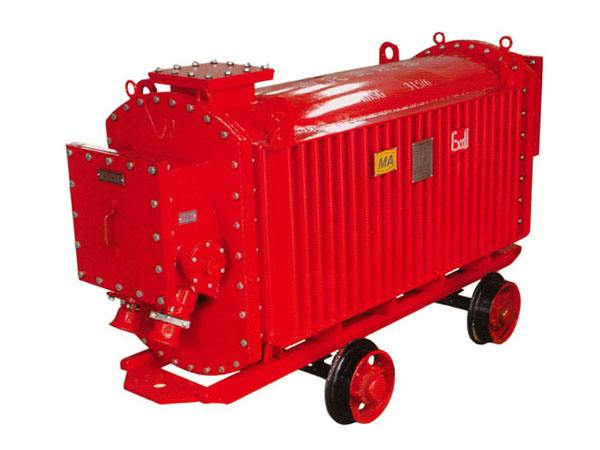15606350552

Email：332333124@qq.com

# 160KVA矿用变压器现货规格

矿用变压器是一种用在矿井下面的变压器类型，它的效率和主要的功能都是比较高的。矿用变压器在使用的过程中也是需要平垫的。平垫是矿用变压器重要的一个部分和结构，那么您知道它是怎么使用的呢?我们和变压器厂家的小编进行详细去了解一下如何进行使用吧：平垫圈简介：

先介绍平垫圈的功能和使用方法，套在螺栓或螺钉上使用，加装平垫圈后，加大接触面积，能分散压力，保护被连接零件表面不被损坏。

平垫圈的安装：

上面介绍了平垫的作用，所以平垫在安装时，要挨着机器/工件的表面。

矿用变压器保护整定计算

a. 过流I段保护

<1>动作电流

1>按躲过低压侧负荷母线发生三相短路时流过装置的大短路电流整定

Iop = Krel*Id.max(3) = 1.4*1701 = 2381.4A

式中 Krel为可靠系数,取1.4

2>按躲过矿用变压器的励磁涌流整定,其动作电流应大于3~5倍额定电流

Iop = 5Ie = 5*1600/(1.732*6.3) = 5*146.63 = 733A

故选取

Iop = 2381.4A

Iop.j = 2381.4/500 = 4.763A

<2>灵敏度校验

按 小方式下保护安装处发生两相短路时流过装置的短路电流

Ksen = Id.min(2)/Iop = 22195*.0866/2381.4 = 8.07

<3>动作时限

取t = 0”

b. 过流

II段保护

<1>动作电流

按躲过矿用变压器低压侧母线发生两相短路时流过的大短路电流整定

Iop = Krel*Id.max(2) = 1.2*0.866*1701 = 1767.68A

Iop.j = 1767.68/500 = 3.54A

<2>动作时限

取t = 0.5”

c. 过流III段保护

<1>动作电流

按躲过低压侧母线上需要自起动的电动机

大起动电流之和整定

取SdΣ = 0.8Se

Kzq = 1/[Ud%+Se/(Kqd*SdΣ)]= 1/[0.08+1/(5*0.8)]= 3.03

Iop = Krel*Kzq*Ie = 1.2*3.03*146.63 = 533.16A

Iop.j = 533.16/500 = 1.066A

式中 Kzq为需要自起动的全部电动机在自起动时所引起的过电流倍数,取5

SdΣ为需要自起

动的全部电动机的总容量

Krel为可靠系数,取1.2

<2>灵敏度校验

按低压侧母线处发生两相金属性短路时流过的 小短路电流

Ksen = Id.min(2)/Iop =0.866*1692/533.16 =2.75

以上是常见的矿用变压器的整定的计算的主要的方法和主要的方式供大家进行参考，对于矿用变压器的其他的操作后续文章中也是会介绍到的，我们和大家进行详细去讲解吧!